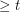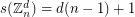# Davenport constant

## Davenport's constant ★★★

Author(s):

For a finite (additive) abelian group, the Davenport constant of, denoted, is the smallest integerso that every sequence of elements ofwith lengthhas a nontrivial subsequence which sums to zero.

ConjectureKeywords: Davenport constant; subsequence sum; zero sum